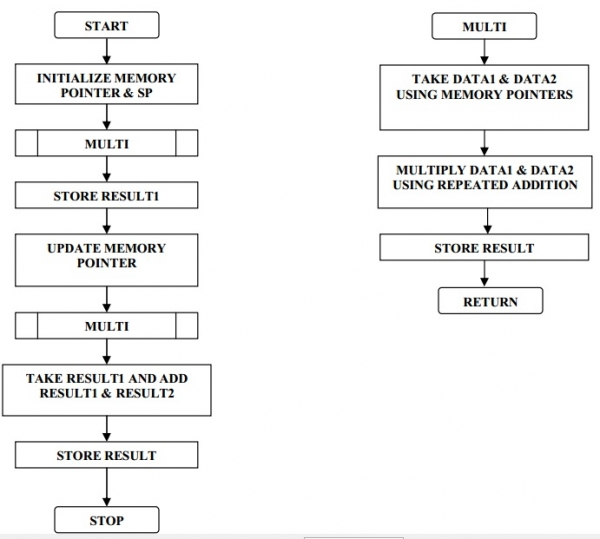# 8085 program to implement the following function (a*b) + (c*d)

Here we will see how to implement mathematical function using 8085.

## Problem Statement

Write a program to implement the following function (a*b) + (c*d) and store the result in memory locations 8204 and 8205. Perform multiplication by using a subroutine. Here a, b, c and d numbers are stored at memory locations 8200H, 8201H, 8202H and 8203 respectively.

## Discussion

Multiplication instruction is not present in the 8085. So we have to use subroutine to perform multiplication. In this subroutine, it takes number from memory pointed by HL pair, and return result into DE register pair. After multiplying two parts, the intermediate results are stored, then add them to get the final result.

Data

8200
02
8201
03
8202
04
8203
05

Data

8200
CA
8201
C6
8202
C8
8203
64

## Flow Diagram## Program

HEX Codes
Labels
Mnemonics
8000
31, 00, 83
START:
LXI SP, 8300 H
Initialize stack pointer SP = 8300 H
8003
21, 00, 82

LXI H, 8200 H
Initialize memory pointer HL = 8200 H which is the starting data block address
8006
CD, 19, 80

CALL MULTI
Call Subroutine Multi.. It takes HL as the source data block pointer and returns result in DE register pair
8009
D5

PUSH D
Store result1 i.e. a * b
800A
23

INX H
Point to next data pair
800B
CD, 19, 80

CALL MULTI
Call Subroutine Multi.. It takes HL as the source data block pointer and returns result in DE register pair
800E
C1

POP B
Get result1in BC which at the top
800F
E5

PUSH H
Store pointer
8010
EB

XCHG
Get result2 in HL i.e. c * d
8011
9

HL= HL+BC (result1+result2: sum of products). So HL = a * b + c * d
8012
EB

XCHG
Store result in DE and HL will be destination pointer
8013
E1

POP H
Restore the memory pointer
8014
23

INX H
8015
73

MOV M, E
Store at HL, LSB of result (HL) = E
8016
23

INX H
Point to the next location
8017
72

MOV M, D
Store at HL, MSB of result (HL)=D
8018
76

HLT
Stop
8019
4E
MULTI:
MOV C, M
C = no1
801A
23

INX H
HL= HL+1; point to next number
801B
46

MOV B, M
B = no2
801C
E5

PUSH H
Save HL register pair
801D
26, 00

MVI H, 00 H
H = 00; to track carry (carry register: will contain the MSB of result)
801F
3E, 00

MVI A, 00 H
A = 00 (Sum register)
8021
80
UP:
A = A + B
8022
D2, 26, 80

JNC LOOP
Is carry = 1? If no go to loop
8025
24

INR H
H = H + 1
8026
0D
LOOP:
DCR C
C = C – 1
8027
C2, 21, 80

JNZ UP
Is Z = 0? If no go to up
802A
6F

MOV L, A
Get sum in L from A (LSB of result)
802B
EB

XCHG
Get result in DE from HL
802C
E1

POP H
Restore back HL register pair
802D
C9

RET
Go back to main

Data

8204
1A
8205
00